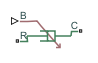# Variable Translational Damper

Translational viscous damper with variable damping coefficient

## Library

Couplings & Drives/Springs & Dampers

•## Description

The block represents a translational viscous damper with variable damping coefficient. A physical signal input port provides the magnitude of the translational damping coefficient. The magnitude of the damping force is equal to the product of the physical signal input and the relative linear velocity between the two translational conserving ports. A minimum damping coefficient prevents non-physical negative damping values.

The translational damping force satisfies the following expression:

`$F=\left\{\begin{array}{cc}B.v,& B\ge {B}_{\mathrm{min}}\\ {B}_{\mathrm{min}}\cdot v,& B<{B}_{\mathrm{min}}\end{array},$`

The parameters are:

• F — Force transmitted through the translational damper between the two translational conserving ports

• B — Viscous damping coefficient

• Bmin — Minimum allowed damping coefficient

• v — Relative linear velocity measured between the two translational conserving ports

• vR — Linear velocity of port R

• vC — Linear velocity of port C

The block applies equal and opposite damping forces on the two translational conserving ports. The sign of the damping force acting on port R is equal to the sign of the relative linear velocity. A positive linear velocity corresponds to a positive damping force acting on port R, and a negative damping force of equal magnitude acting on port C.

### Assumptions and Limitations

• The block represents strictly viscous damping.

• The value of the damping coefficient must be greater than or equal to zero.

## Ports

`B`

Physical signal input port representing the variable translational damping coefficient.

`C`

Translational conserving port.

`R`

Translational conserving port.

## Parameters

Minimum damping coefficient

Minimum value allowed for the variable damping coefficient. The physical signal input saturates below the specified value. The parameter must be greater than or equal to zero. The default value is `0` `N/(m/s)`.

## Version History

Introduced in R2013a